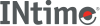﻿ fcvtINtime SDK Help
fcvt

Converts a double value to a null-terminated string, indicating the sign and decimal point location.

```#include <stdlib.h>

char *fcvt (double value, int count, int *dec, int *sign);
```

#### Parameters

`value`
Value to convert. Stores the digits of value as a string and appends a null character \0.
`count`
Number of digits to store after decimal point. Excess digits are rounded off to count places. If there are fewer than count digits, the string is padded with 0s.
`dec`
Points to an integer value, which gives the position of the decimal point with respect to the beginning of the string. A 0 or negative integer value indicates that the decimal point lies to the left of the first digit.
`sign`
Points to an integer indicating the sign of value.
 0 Positive Not 0 Negative

#### Remarks

Only digits are stored in the string. Obtain the position of the decimal point and the sign of value from dec and sign after the call.

fcvt uses a single statically allocated buffer for the conversion. Each call destroys the results of the previous call.

#### Return Values

A pointer to the string of digits.

#### Requirements

Versions Defined in Include Link to
INtime 3.0 intime/rt/include/stdlib.h (or)
intime/rtinclude/math.h
stdlib.h (or)
math.h
clib.lib